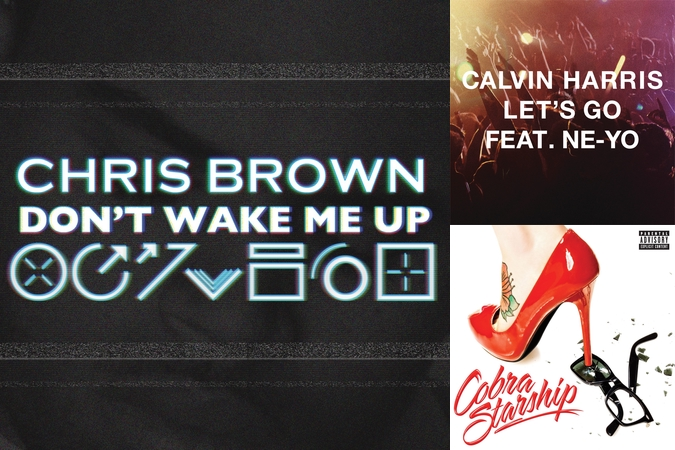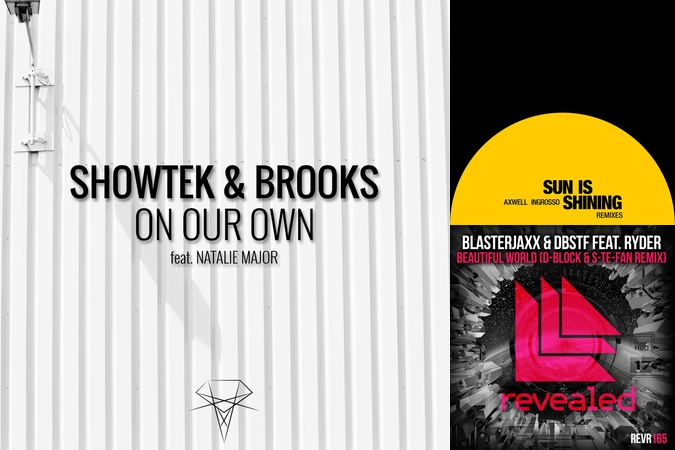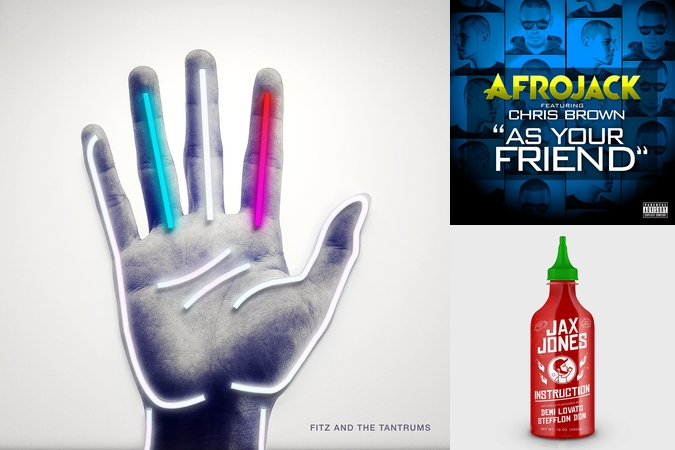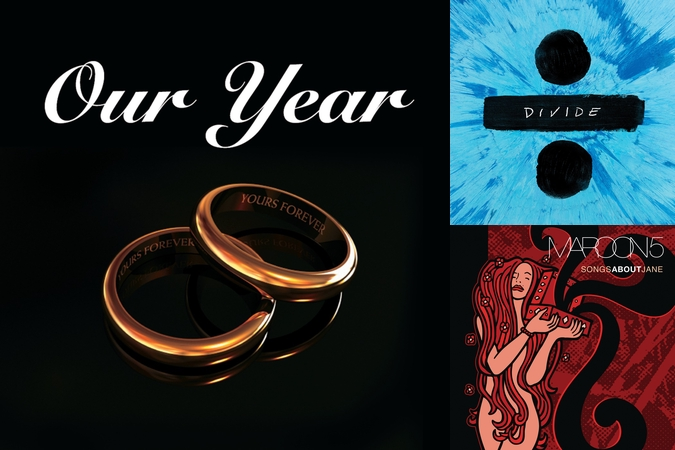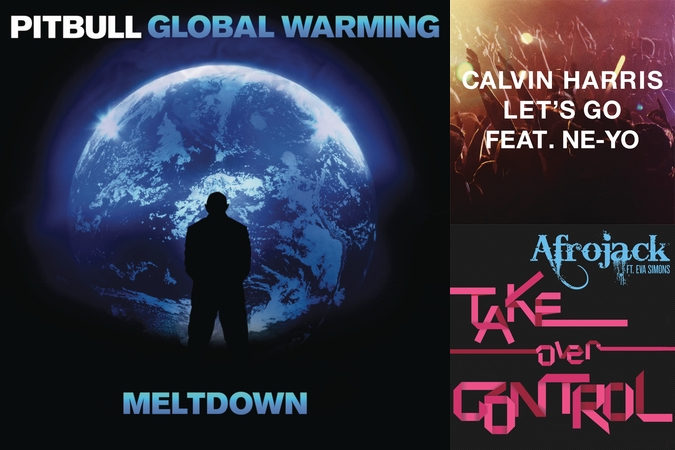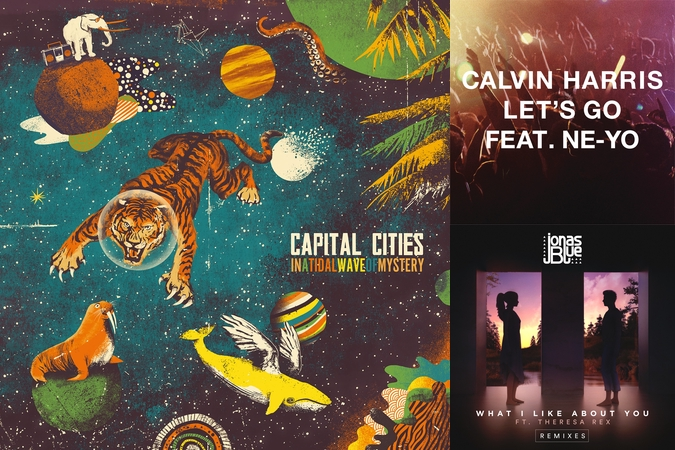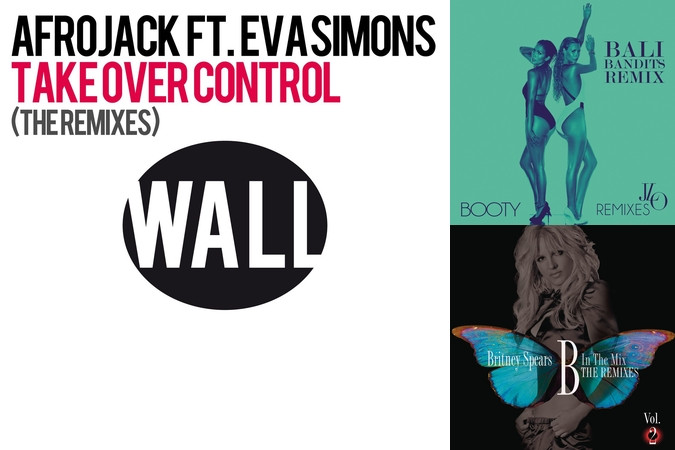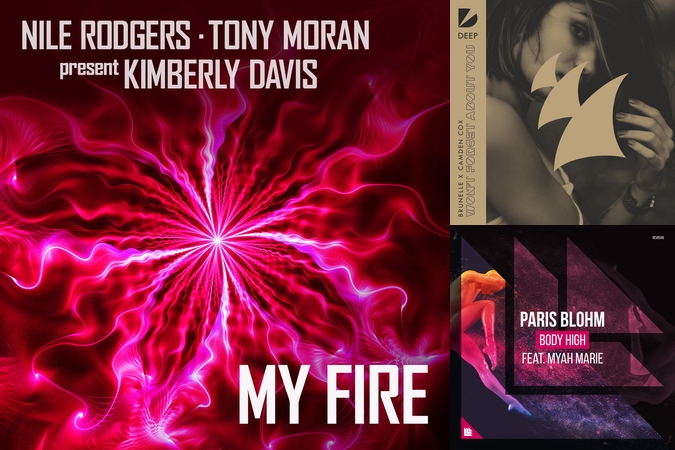## Track by Calvin Harris

78,327
5,972

ALBUM TRACKS
LYRICS

let's go make no excuses now i'm talking here and now i'm talking here and now let's go your time is running out i'm talking here and now i'm talking here and now it's not about what you've done it's about what you doing it's all about where you going no matter where you've been let's go let's go let's go let's go let's go let's go there ain't no better time i'm talking here and now i'm talking here and now let's go right now is where you shine i'm talking here and now i'm talking here and now it's not about what you've done it's about what you doing it's all about where you going no matter where you've been let's go let's go let's go let's go let's go let's make it happen ooh let's make it happen tonight let's make it happen ooh let's make it happen tonight let's make it happen ooh let's make it happen tonight let's make it happen ooh let's make it happen tonight let's go let's go let's go let's go let's go

APPEARS ON PLAYLISTS